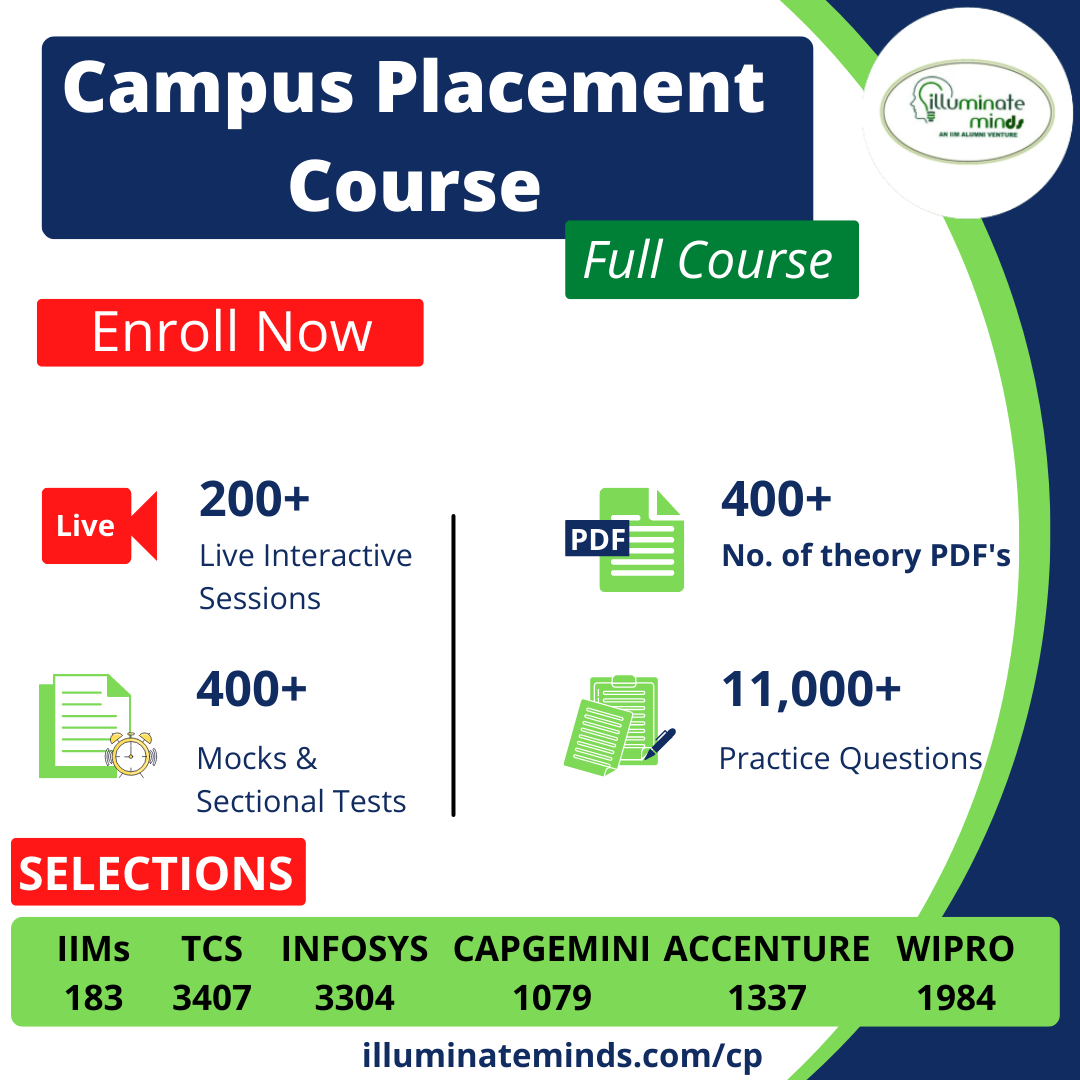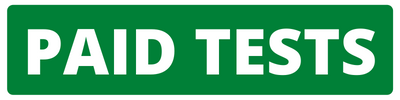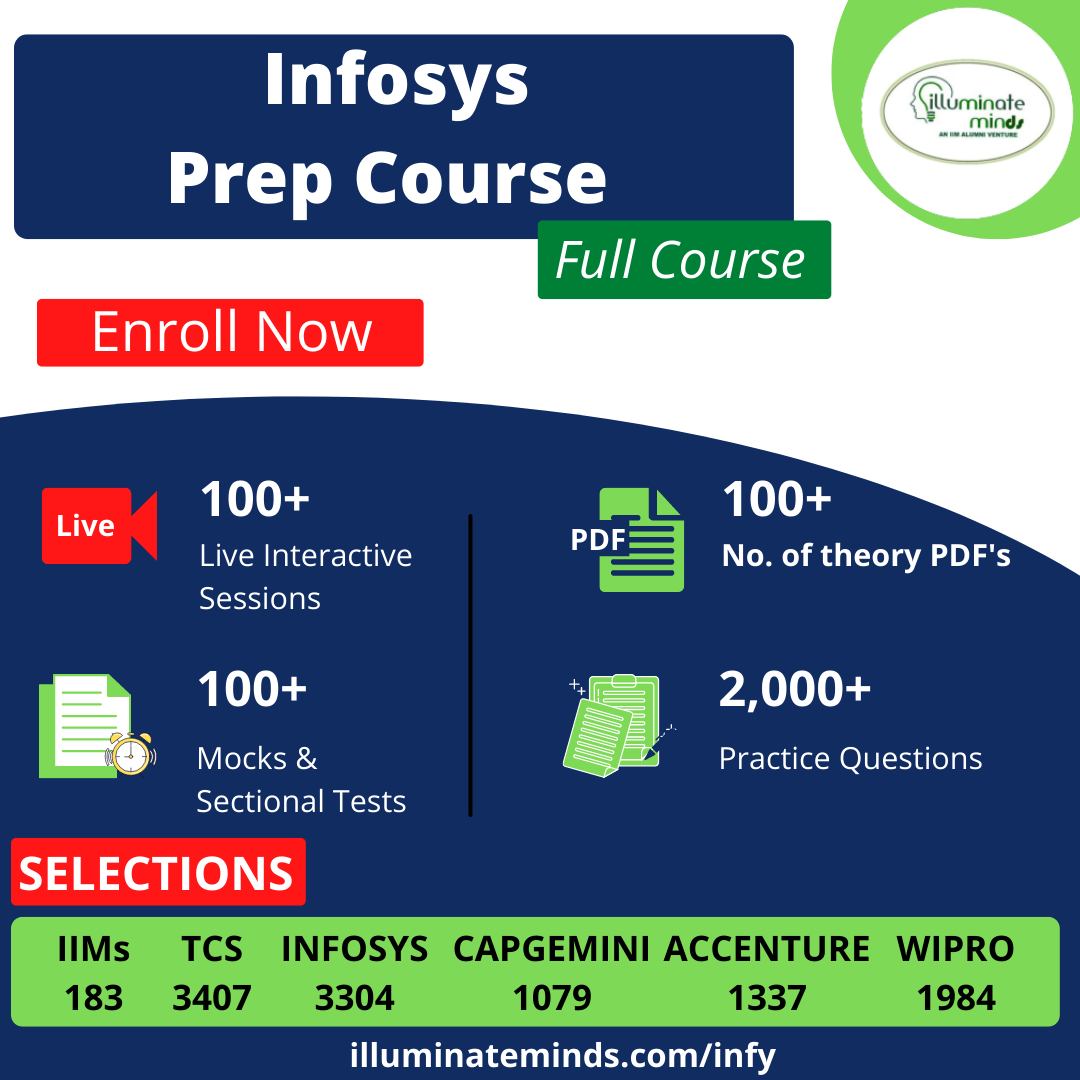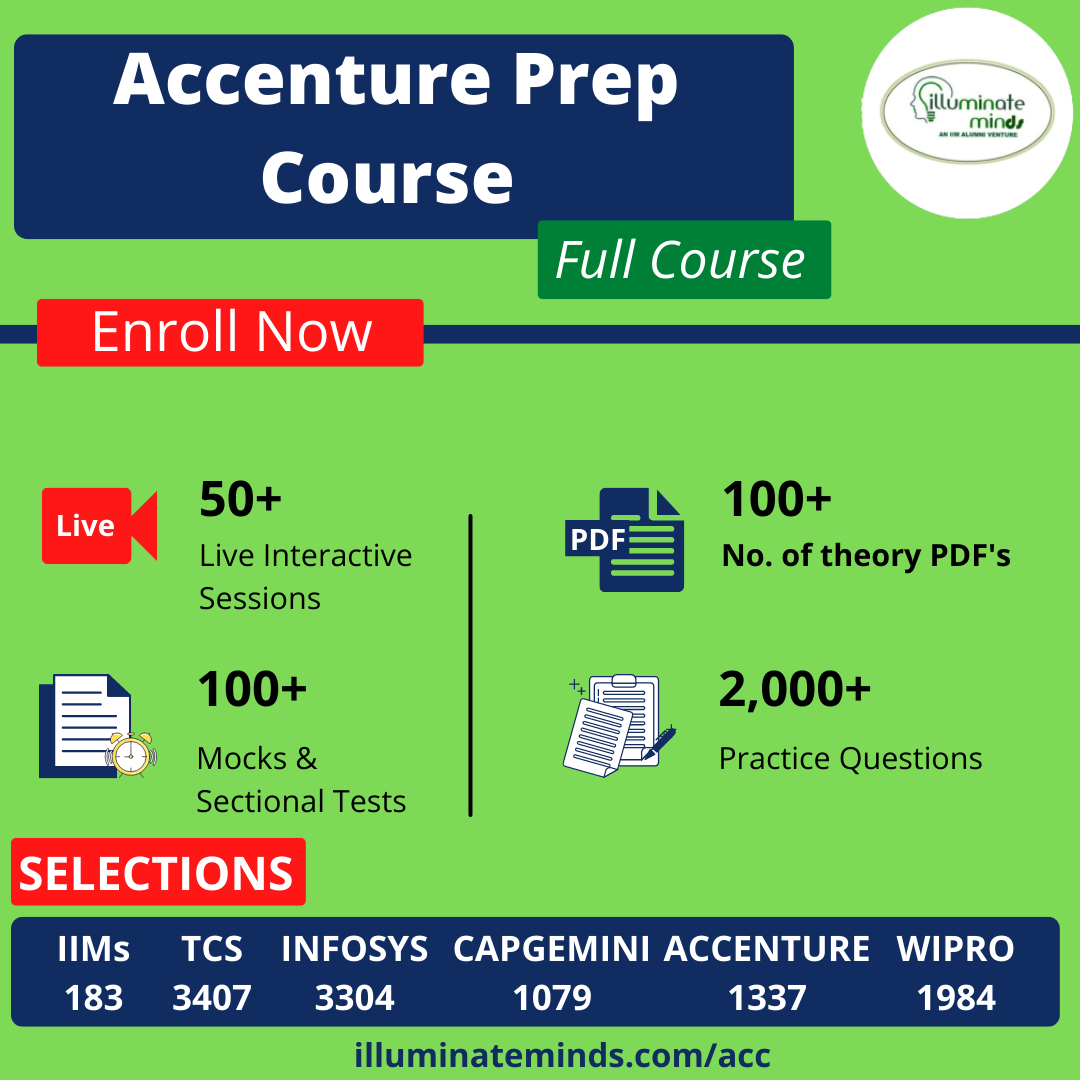CAT | AIMCAT Quants Questions

## AIMCAT Quants QuestionsJobs By Batch
Jobs By Location
Jobs By Degree
Jobs By Branch
IT Jobs
Internships
Govt. Jobs• ##### Original Questions of IT Companies| All Topics### Quiz Begins Here

Q #1
:

#### AIMCAT Quants Question

Which smallest number should be multiplied by 45 so that it will have 3 distinct prime factors?
+

Prime factors of 45 are 3 x 3 x 5 i.e. 3 and 5.

The smallest number to be multiplied by 45 so that it may have 3 distinct prime factors is 2.

Number become 90 (45x2) and factors are 2x3x3x5.

Q #2
:

#### AIMCAT Quants Question

A, B and C are three students who attend the same tutorial classes. If the probability that on a particular day exactly one out of A and B attends the class is 710; exactly one out of B and C attends is 410; exactly one out of C and A attends is 710. If the probability that all the three attend the class is 9100, then find the probability that at least one attends the class.
+

Probability of at least one attending the class = 1- probability of none attending

Let probability of A, B and C attending the class be a, b, c respectively.

Thus, probability of not attending the class by A, B and C is (1 - a), (1 - b) and (1 – c) respectively.

The probability of exactly one of A or B attending = a(1 - b) + b(1 - a) = 710

= a + b – 2ab = 710 (1)

Similarly, for B or C = b + c – 2bc = 410 (2)

And, for C or A = c + a – 2ca = 710 (3)

Probability of all three attending (i.e. abc) = 99100 (4)

From (1), (2) and (3): 2(a + b + c – ab – bc - ca) = 710 +410 + 710 = 1810 = 95

a + b + c – ab – bc – ca = 910

Probability of none attending = (1 - a)(1 - b)(1 – c) = 1 – a – b – c + ab + bc + ca – abc

Probability of none attending = 1 – (a + b + c – ab – bc – ca + abc)

Probability of none attending = 1 – (910 + 99100) = 1100

Probability of at least one attending = 1 – probability of none attending

Probability of at least one attending = 1 – 1100 = 99100

Q #3
:

#### AIMCAT Quants Question

A bag is full of 20 bananas and no other fruit. Rajeev draws a fruit from the bag. What is the probability that he will draw a banana?
+

As any drawn fruit will be a banana so the probability of drawing a banana is 1

Q #4
:

#### AIMCAT Quants Question

A shopkeeper offers �Buy 1, Get 1 Free� offer on a T � shirt marked at Rs. 2, 400. If after a sale, the shopkeeper earns a profit of 33.33% then what is the actual price of the T- shirt?
+

The shopkeeper sold two T-shirts for Rs. 2400

Selling Price of one T-shirt = Rs. 1200

Profit = 33.33%

Cost Price = S.P. * 100100+P% = 1200 * 100100+33.33 = 1200 * 100133.33 = 1200 * 34 ≈ Rs. 900

Q #5
:

#### AIMCAT Quants Question

What is the value of the expression 423 * 520 * 6-2 * 32 * 5-5 * 2-46 * 5-10 * 110 * 5-5?

+

423*520*32*11062*55*246*510*55 = (22)233*520*32*1(3*2)2*55+10+5*246 = 246*520*32*122*32*520*246 = 122 = 14